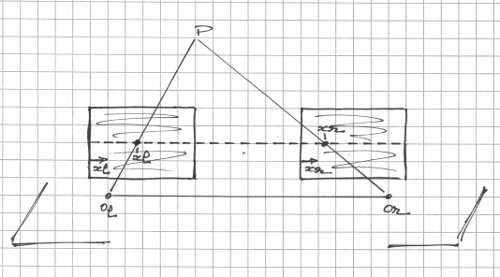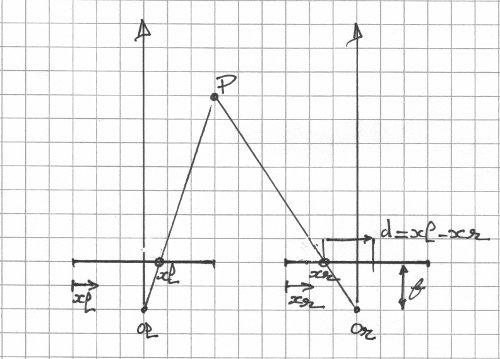## Monday, January 2, 2012

### Stereo Matching - Rectified Geometry

In general, in stereo photography, the image planes (image sensors in digital photography) are not necessarily co-planar and aligned with each other, among other things. Rectification remedies this problem with the end result being that a point in space projects to two location on the same scan line (same row, if you will) in the left and right camera images.Rectified stereo geometry (3d view).

In the diagram above, O_l and O_r are the optical centers (lens centers) for the left and right lenses. Each image plane defines a two-dimensional coordinate system: a pixel in the left image is defined by its coordinates (x_l,y_l) and a pixel on the right image is defined by its coordinates (x_r,y_r). The point P projects to (x_l,y_l) on the left image plane and (x_r,y_r) on the right image plane such that y_l=y_r=y. The line (row) at ordinate y is a scan line.

In reality, the image planes are positioned behind the optical centers (at f, where f is the focal length) but placing them in front makes it easier because you don't have to deal with image inversion.

If you consider the plane (O_l,O_r,P), the image planes are reduced to the scan line:Rectified stereo geometry (scan line view).

The vertical lines emanating from the optical centers are the optical axes (lens axes) - they are exactly parallel to each other. The disparity for point P is defined as d=x_l-x_r. Once you know the disparity of a point, geometry of the stereo camera (focal length and baseline, the distance between the two optical centers) gives its depth in the scene.

Dense stereo matching (or correspondence) consists in finding the disparity for every pixel in the left and/or right image (depth map). It is a difficult problem for many reasons (we will look into those in turn in future posts). When the stereo images are rectified, the complexity of stereo matching is slightly reduced (the hard part resides elsewhere).

1.2.